Question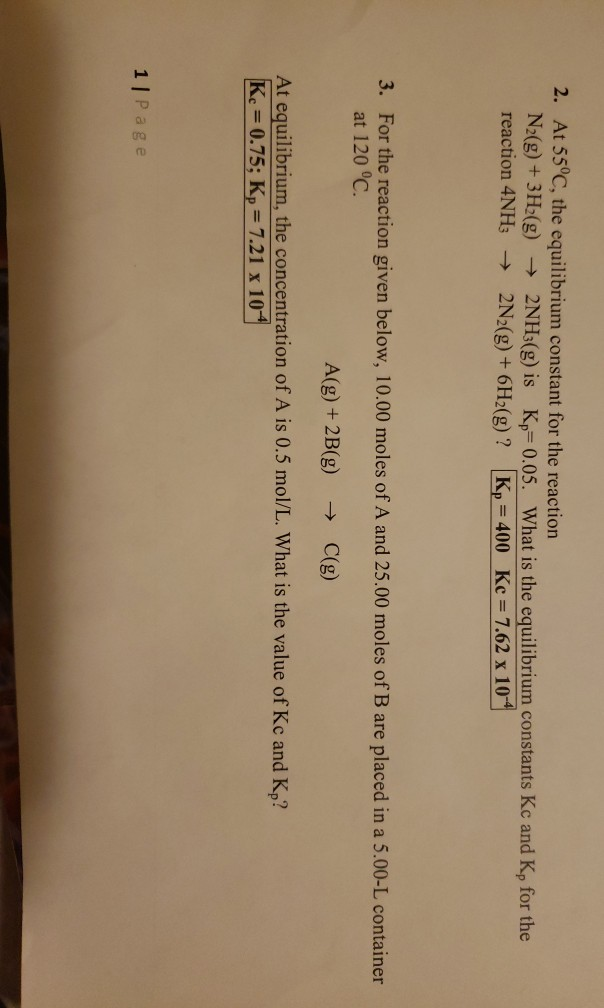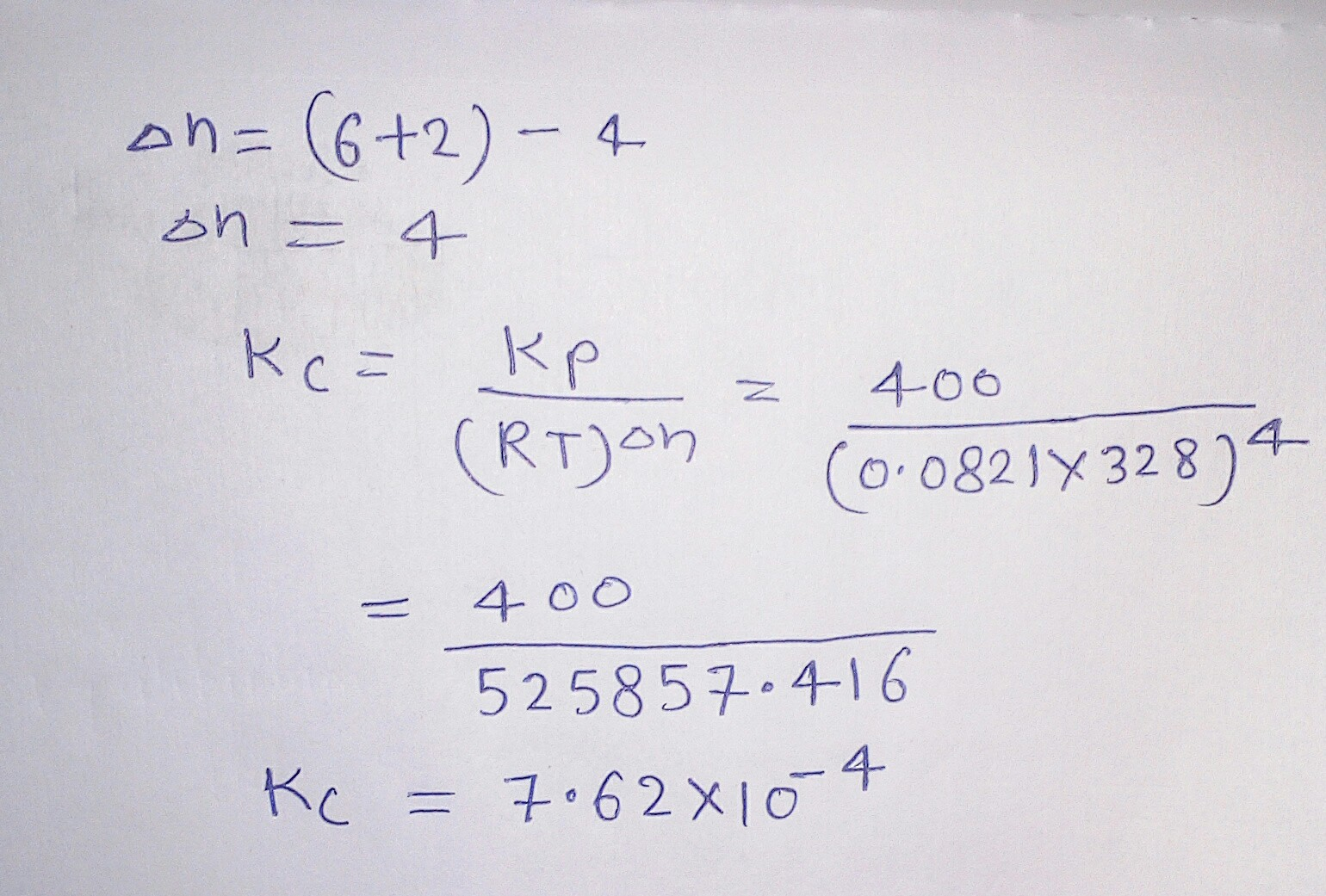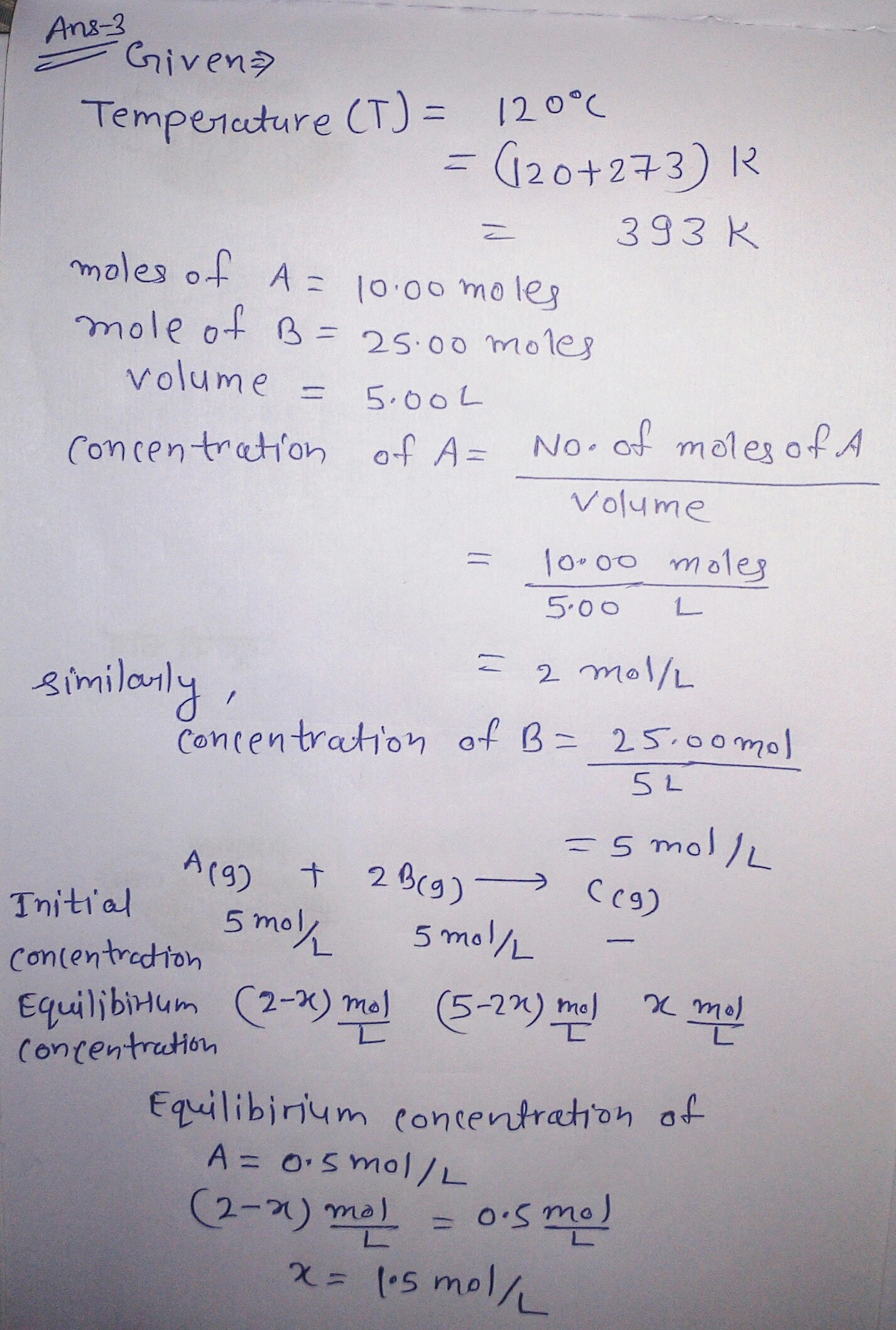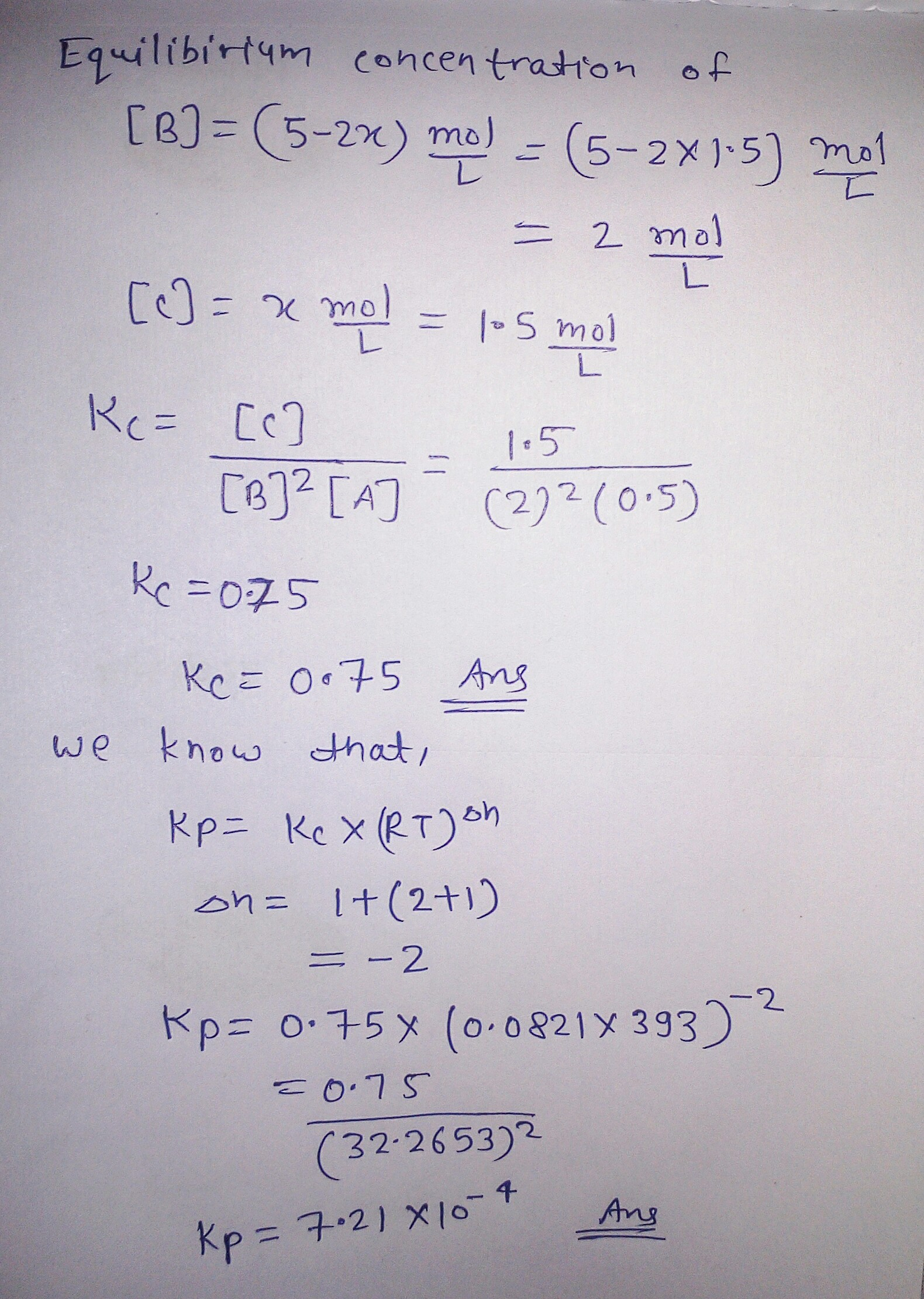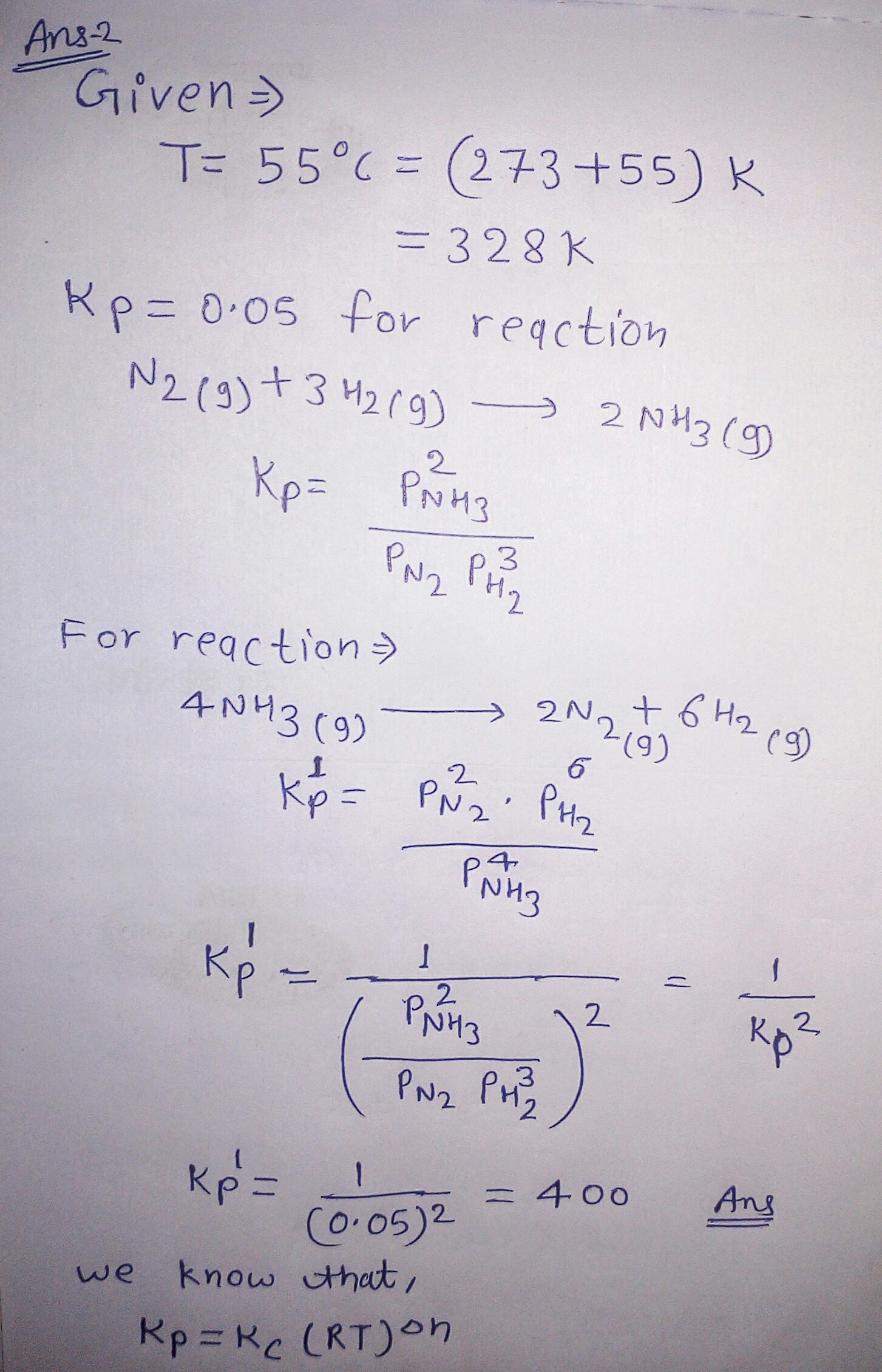#### Earn Coins

Coins can be redeemed for fabulous gifts.

Similar Homework Help Questions
• ### The equilibrium constant for the chemical equation N2(g)+3H2(g)−⇀↽−2NH3(g) is Kp=0.111 at 231 ∘C. Calculate the value...

The equilibrium constant for the chemical equation N2(g)+3H2(g)−⇀↽−2NH3(g) is Kp=0.111 at 231 ∘C. Calculate the value of Kc for the reaction at 231 ∘C.

• ### Consider the chemical reaction 2NH3(g) ó N2(g) + 3H2(g). The equilibrium is to be established in...

Consider the chemical reaction 2NH3(g) ó N2(g) + 3H2(g). The equilibrium is to be established in a 50.0 L container at 1,000 K, where Kc = 4.0 × 10-2. Initially, 6.10 x 105 moles of NH3(g) are present. Calculate the amount of H2 presentat equilibrium. [H2] =_____

• ### The equilibrium constant, Kc, for the following reaction is 6.30 at 723K. 2NH3(g) N2(g) + 3H2(g)...

The equilibrium constant, Kc, for the following reaction is 6.30 at 723K. 2NH3(g) N2(g) + 3H2(g) If an equilibrium mixture of the three gases in a 15.7 L container at 723K contains 0.284 mol of NH3(g) and 0.437 mol of N2, the equilibrium concentration of H2 is__________ M.

• ### Enter your answer in the provided box. For the reaction N2(g) + 3H2(g)⇆ 2NH3(g) KP is...

Enter your answer in the provided box. For the reaction N2(g) + 3H2(g)⇆ 2NH3(g) KP is 0.000205 at 563°C. What is Kc for the reaction? Kc =

• ### For the reaction N2(g)+3H2(g)⇌2NH3(g) Kp = 3.10×10−3 at 323 ∘C . What is Kc for the...

For the reaction N2(g)+3H2(g)⇌2NH3(g) Kp = 3.10×10−3 at 323 ∘C . What is Kc for the reaction at this temperature? Enter your answer numerically. Kc= ?

• ### Consider this reaction: N2(g) + 3H2(g) <==> 2NH3(g) If the Kc for the reaction is 4.5...

Consider this reaction: N2(g) + 3H2(g) <==> 2NH3(g) If the Kc for the reaction is 4.5 x 10^4 at 127 degrees celsius, what is the equilibrium constant, Kp at the same temperature?

• ### For the reaction N2(g)+3H2(g)⇌2NH3(g) Kp=2.55×10−3 bar−2 at 330 ∘C . What is Kc for the reaction...

For the reaction N2(g)+3H2(g)⇌2NH3(g) Kp=2.55×10−3 bar−2 at 330 ∘C . What is Kc for the reaction at this temperature?

• ### 4. At a given temperature, K = 1.44 × 10-2 for the reaction: N2(g) + 3H2(g)...

4. At a given temperature, K = 1.44 × 10-2 for the reaction: N2(g) + 3H2(g) ⇌ 2NH3(g) Calculate values of K for the following reactions at the given temperature. a. 4NH3(g) ⇌ 2N2(g) + 6H2(g) b. ½N2(g) + ³∕2H2(g) ⇌ NH3(g)

• ### Enter your answer in the provided box. For the reaction N2(g) +3H2(g)* 2NH3(g) Kp is 0.000667...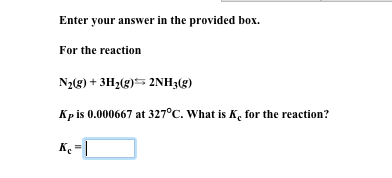Enter your answer in the provided box. For the reaction N2(g) +3H2(g)* 2NH3(g) Kp is 0.000667 at 327°C. Wha is Kc for the reaction?

• ### The equilibrium constant, K, for the following reaction is 6.30 at 723K. 2NH3(g) N2(g) + 3H2(g)...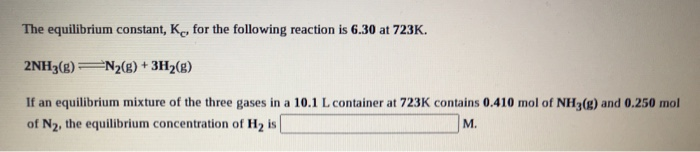The equilibrium constant, K, for the following reaction is 6.30 at 723K. 2NH3(g) N2(g) + 3H2(g) If an equilibrium mixture of the three gases in a 10.1 L container at 723K contains 0.410 mol of NH3(g) and 0.250 mol of N2, the equilibrium concentration of Hy is M.